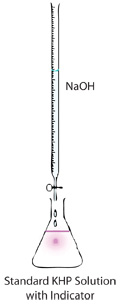# Exp 2: Volumetric Analysis

-Summary

-Evaluation Questions: Virtual Titrations

Experiment 2 Virtual Lab Tutorial >> Acid Base Titration >> Evaluation Question

 Next >

# Experiment 2 Virtual Lab Tutorial: Acid Base Titration

## Summary (from the Lab Manual and the Techniques Tutorial)

Volumetric analysis (VA) is a quantitative analytical process based on measuring volumes. The most common form of VA is the titration, a process whereby a standard solution of known concentration is chemically reacted with a solution of unknown concentration in order to determine the concentration of the unknown. In the first part of this experiment KHP (the standard) is reacted with sodium hydroxide (concentration unknown) until the reaction between the two compounds reaches completion (called the "end-point" of the titration). The endpoint occurs when the first pale pink colour appears in the colourless solution and stays (upon swirling) for 30 - 60 seconds. Once the sodium hydroxide solution has been standardized, it can then be used to standardize a hydrochloric acid solution of unknown concentration.Measurements in VA must be have at least 4 significant digits. As you perform each step in this experiment, think about whether every instrument you use fulfills this requirement. Make sure that you maintain this number of significant figures when transferring reagents from one container to another.

• Each titration will be performed on a 25.00mL sample of solution (the 25.00 mL pipet is the only one available in the lab). Think about the following requirements to ensure 4 significant figures in the titrations:

• The volume of the titrant used in the titration should be at least 10.00mL (why?)

• In a titration, best results are obtained when similar volumes of the titrant and titrated sample are used (what would be an ideal volume of the titrant?)

• The capacity of a buret is 50.00mL. If the buret needs to be re-filled during the titration, an additional opportunity for introducing an error is created.

• If the concentrations of the titrant and the titrated sample are very different, and a 25.00mL sample is used, how would you ensure that the volume of the titrant is about 25 mL?

• If the solution of the unknown (HCl) needs to be diluted, the dilution should be performed with quantitative precision (using a pipet and a volumetric flask). Why?

• If the solution of the NaOH needs to be diluted for the purpose of standardization with the KHP standard, that dilution should be done with a graduated cylinder (quantitative dilution is not required) Why?

The next pages contain the virtual lab as part of an interactive feedback activity. Please think about the above questions and click 'next' to continue to the activites.

 Next >